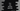# How to use ternary operator in C++## How to use ternary operator in C++:

Ternary operator is also called conditional operator. It is similar to if-else statement, but we can use this to write a condition in just one line. Ternary opeartor is really helpful if you need to check for a condition quickly in one line.

In this post, we will learn how to use ternary operator in C++ with examples.

## Syntax of ternary operator:

Below is the syntax of the ternary operator in C++:

``result = condition ? first : second;``

Here, the value of result is calculated by using the condition. If the value of condition is true, it will assign first to result. Else, it will assign second to result.

first and second can be expressions or values.

If I write this syntax for if-else, it will look as like below:

``````if(condition){
result = first;
}else{
result = second;
}``````

As you can see here, it is actually same as if-else statement. Now let’s try some examples:

## Example 1: ternary operator:

Let’s take a look at the below example:

``````#include <iostream>
using namespace std;

int main()
{
int i;

cout << "Enter the value of i: " << endl;
cin >> i;

string result = i % 2 == 0 ? "It is even" : "It is odd";

cout << result << endl;

return 0;
}``````

In this example,

• i is an integer value and we are taking the value of i as an input from the user.
• If the value of i % 2 == 0, it assigns It is even to result. Else, it assigns It is odd to result.
• The last line is printing the value of result.

If we run this program, it will print output as like below:

``````Enter the value of i:
10
It is even

Enter the value of i:
11
It is odd``````

## Example 2: ternary operator with expression:

Let’s try ternary operator with expressions. We can assign the result of an expression using ternary operator. For example:

``````#include <iostream>
using namespace std;

int main()
{
int i;

cout << "Enter the value of i: " << endl;
cin >> i;

int result = i % 2 == 0 ? i * 10 : i * 20;

cout << result << endl;

return 0;
}``````

Here, we are assigning i * 10 to result if i is even. Else, we are assigning i * 20. You can also use any function call as well.